# ggplot2 in R

Today we’ll be learning about one of the most popular packages in R and which is ggplot2: An Implementation of the Grammar of Graphics. I’m using iris data set which is available in the R. ggplot2 create plot in multiple layers. I’ve used ggplot to create correlation heatmap. I’ve provided more resources in the end of the blog.

The structure of ggplot looks:
`ggplot (data = data used for plot, aes=(mappings)) + geom_functions `

geom_function is the layer used to create the plot.

we’ll see the detailed description with the examples.

First we’ll install the load the ggplot2 package in R using the following commands:
```install.packages("ggplot2")
library(ggplot2)

```

We’ll use iris data for further analysis. Let’s check the data first
```> head(iris)

Sepal.Length Sepal.Width Petal.Length Petal.Width Species

1          5.1         3.5          1.4         0.2  setosa

2          4.9         3.0          1.4         0.2  setosa

3          4.7         3.2          1.3         0.2  setosa

4          4.6         3.1          1.5         0.2  setosa

5          5.0         3.6          1.4         0.2  setosa

6          5.4         3.9          1.7         0.4  setosa

```

●  We’ll start plotting the scatter plot between sepal length and sepal width for the species.
```Plot  <-  ggplot(data= iris, aes(Sepal.Length, Sepal.Width))
+ geom_point()```
So here data  components takes iris data set, aes takes sepal length and sepal width as x and y values for the plot and the geom_point() to plot scatter plot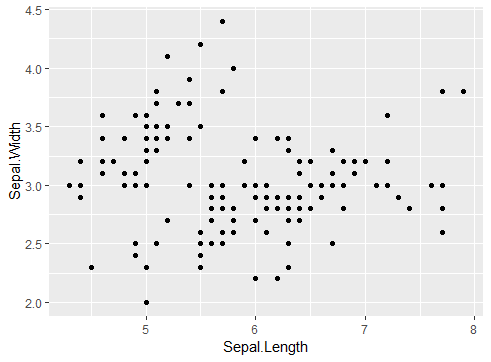Scatter Plot

●  Let’s color the points for different species by adding color in aes.
```Plot1 <-  ggplot(data= iris, aes(Sepal.Length, Sepal.Width,color=Species))
+ geom_point()```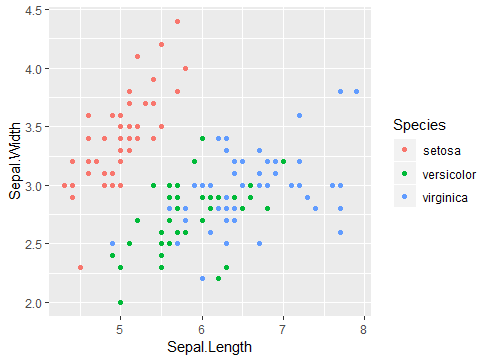Scatter Plot with color

●  Line Plot
```Plot2 <- ggplot(data= iris, aes(Sepal.Length, Sepal.Width))
+ geom_line()```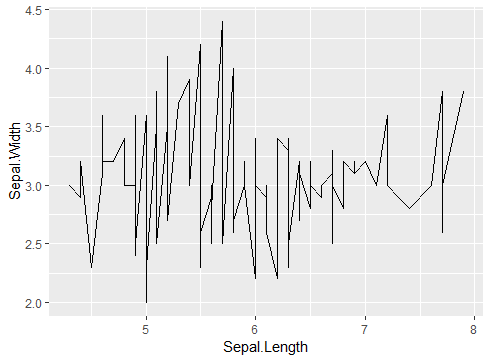Line Plot

●  Dual Axis- Plotting line and scatter plot together.
```Plot3 <- ggplot(data= iris, aes(Sepal.Length, Sepal.Width,color=Species))
+ geom_point() + geom_line()```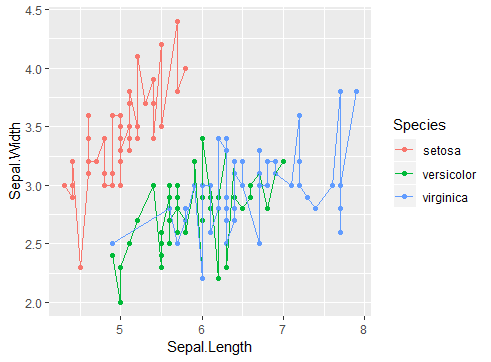Dual Axis Plot

●  Smoothing fit Model in ggplot
```Plot4 <- ggplot(data= iris, aes(Sepal.Length, Sepal.Width,color=Species))
+ geom_point() + geom_smooth(method ="lm")```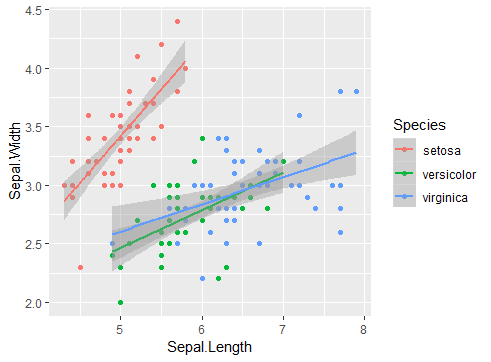Smoothing Model Plot

●  Lets have different scatter plot for different species. Facets divide a plot into subplots based on the values of one or more discrete variables.
```Plot5<- ggplot(data= iris, aes(Sepal.Length, Sepal.Width,color=Species))
+ geom_point()+ facet_wrap(~Species, nrow=2, ncol=2)```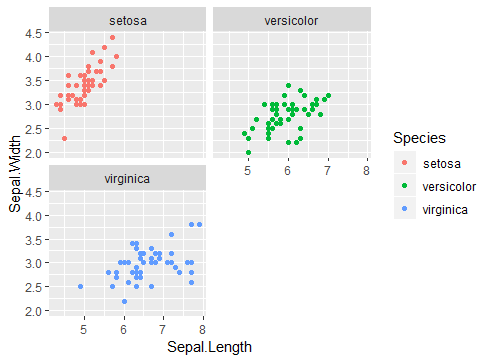Facet Wrap in ggplot

##### Extensions to ggplot2

There are few more packages which are extension of ggplot2. We’ll see ‘GGally’ and gridExtra package.

GGally– Plot all possible relations from the dataset using GGally using the following command:

```install.packages("GGally")
library(GGally)
ggpairs(iris)```

As we can see in the image below ggpair is plotting all possible relations in the available data set. Can be a starting for doing EDA but be careful if dataset is large it can take long time to produce the result. I’ve provide documentation link the resource section below.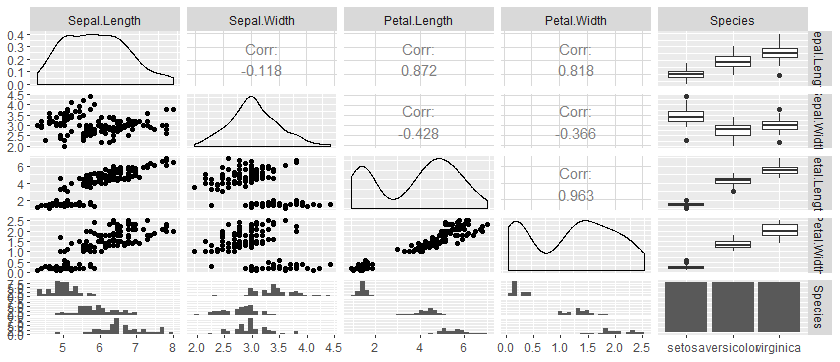ggpair

gridExtra– Using gridExtra we can combine multiple plots in single output window just like a dashboard easy to use and apply. Using the following command.

```install.packages("gridExtra")
library(gridExtra)

#grid.arrange(list of plots to combine, nrow, ncol)
grid.arrange(Plot1, Plot3, ncol=2)```gridExtra

##### Further readings and resources:

Keep visiting Analytics Tuts for more tutorials.

Thanks for reading! Comment your suggestions and queries.